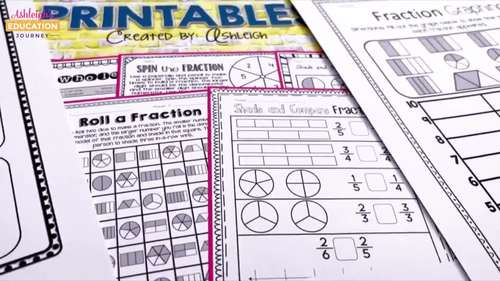# No Prep Fractions Printables | Print and Digital

Rated 4.82 out of 5, based on 2237 reviews
2.2k Ratings;
3rd - 4th, Homeschool
Subjects
Resource Type
Standards
Formats Included
• PDF
•Google Apps™
Pages
Report this resource to TPTThe Teacher-Author indicated this resource includes assets from Google Workspace (e.g. docs, slides, etc.).

#### What educators are saying

This was an excellent resource for teaching fractions. I like that it had both print and digital options.
This this product! There are so many resources and ways to help students practice and understand fractions!
##### Also included in
1. FUN Math PracticeGive your students FUN math practice with these 300+ printables! This bundle contains all six of my no prep math pacts: addition and subtraction, multiplication, division, fractions, area and perimeter, and measurement.****************************************************************
Price \$38.60Original Price \$49.25Save \$10.65

### Description

Give your students FUN fraction practice! These fraction worksheets include equal parts, identifying fractions, fractions in a set, fractions on a number line, comparing fractions, and equivalent fractions.

No Prep Needed

All you have to do is print, and you're ready to go! These fraction printables are a great way to supplement your existing math curriculum. The interactive worksheets are designed to be student and teacher friendly, because no one wants boring practice!

These worksheets were designed for third grade, but can be used with fourth grade students who need additional fraction review.

What's Included

Vocabulary

Pgs. 3-4-Vocabulary Booklet

Equal Parts

Pg. 5 Equal or Not

Pg. 6 Partition the Fraction

Unit Fractions

Pg. 7 Color the Unit Fraction

Pg. 8 Draw a Unit Fraction

Basic Fractions

Pg. 9 Parts of a Whole

Pg. 10 Color the Fraction

Pg. 11 Partition and Color the Fraction

Pg. 12 Identify the Fraction

Pg. 13 Draw a Fraction

Pg. 14 Fractions in a Set

Pg. 15 Identify the Fraction in a Set

Pg. 16 Draw the Fraction in a Set

Pg. 17 Fraction Graphing

Pg. 18 Label the Fraction Bars

Pg. 19 Fraction Word Problems

Pg. 20 Cut and Paste Fractions

Pg. 21 Roll a Fraction

Pg. 22 Spin the Fraction

Fractions on a Number Line

Pg. 23 Missing Numbers on Number Lines

Pg. 24 Name the Fraction

Pg. 25 Mark the Fractions

Pg. 26 Number Line Cut and paste

Fractions Mixed Representations

Pg. 27 Representing Fractions 1

Pg. 28 Representing Fractions 2

Pg. 29 Understanding Fractions

Whole Numbers

Pg. 30 Fractions as Whole Numbers

Pg. 31 Whole Number Graphing

Pg. 32 Cut and Paste Whole Numbers

Pg. 33 Spin and Color

Pg. 34 Fraction Bump

Equivalent Fractions

Pg. 35 Equivalent Fractions

Pg. 36 Label Equivalent Fractions-halves

Pg. 37 Label Equivalent Fractions-thirds

Pg. 38 Label Equivalent Fractions-fourths

Pg. 39 Shade and Name Equivalent Fractions-Rectangles

Pg. 40 Shade and Name Equivalent Fractions-Circles

Pg. 41 Missing Number

Pg. 42 Equivalent Fraction Graphing

Pg. 43 How Many Ways

Pg. 44 Spin and Color

Comparing Fractions

Pg. 45 Comparing Fractions With Bars

Pg. 46 Comparing Fractions With Circles

Pg. 47 Comparing Fractions With Number Lines

Pg. 48 Comparing Fractions

Pg. 49 Shade and Compare Fractions

Pg. 50 Comparing Fractions Same Numerator-No Model

Pg. 51 Comparing Fractions Same Denominator-No Model

Pg. 52 Comparing Fraction Word Problems

Pg. 53 Roll and Compare

Pg. 54 Comparing Fractions Game

Pg. 55-92

3.NF.1

3.NF.2

3.NF.3

Total Pages
Included
Teaching Duration
N/A
Report this resource to TPT
Reported resources will be reviewed by our team. Report this resource to let us know if this resource violates TPT’s content guidelines.

### Standards

to see state-specific standards (only available in the US).
Understand a fraction 1/𝘣 as the quantity formed by 1 part when a whole is partitioned into 𝘣 equal parts; understand a fraction 𝘢/𝑏 as the quantity formed by 𝘢 parts of size 1/𝘣.
Understand a fraction as a number on the number line; represent fractions on a number line diagram.
Represent a fraction 1/𝘣 on a number line diagram by defining the interval from 0 to 1 as the whole and partitioning it into 𝘣 equal parts. Recognize that each part has size 1/𝘣 and that the endpoint of the part based at 0 locates the number 1/𝘣 on the number line.
Represent a fraction 𝘢/𝘣 on a number line diagram by marking off 𝘢 lengths 1/𝘣 from 0. Recognize that the resulting interval has size 𝘢/𝘣 and that its endpoint locates the number 𝘢/𝘣 on the number line.
Explain equivalence of fractions in special cases, and compare fractions by reasoning about their size.